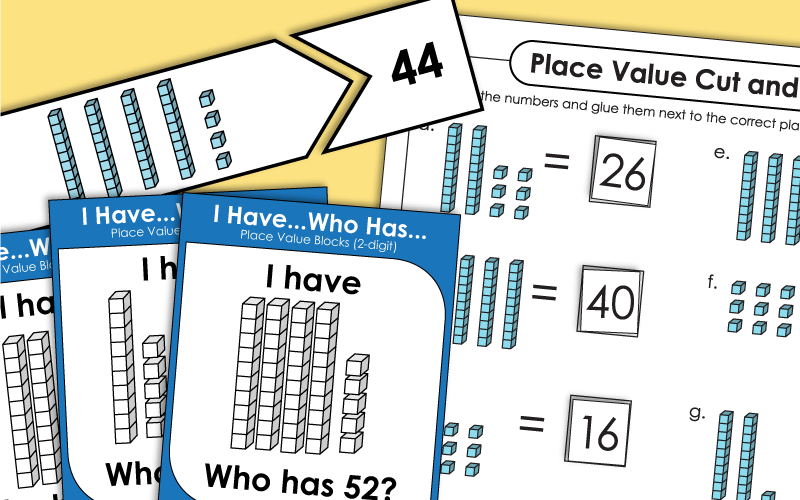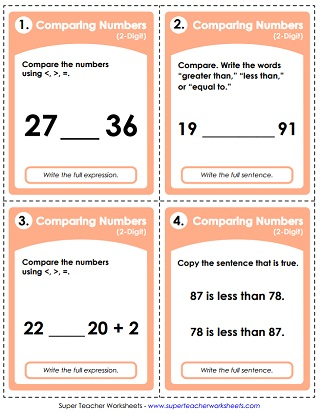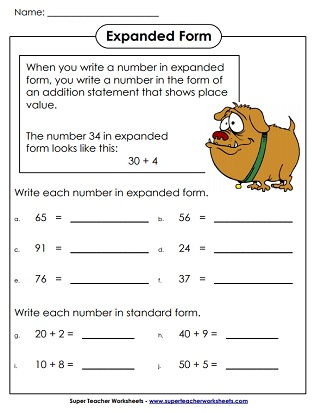# Place Value: 2-Digit Numbers

Use these printable worksheets and games to help teach 2-digit place value. Skills include expanded form, determining the value of the underlined digit, reading numbers, and ordering numbers. These activities are designed for students in Kindergarten through 2nd grade.## Place Value

Cut out the number boxes and paste them next to the correct place value blocks.
Match the number box on the bottom of the page to the correct tens and ones blocks.
Roll two dice and make a 2-digit number. Then write the number in expanded form. After that, draw a sketch of place value blocks that make the number.
Write the 2-digit number represented by each set of place value blocks.
This whole-class "I have, Who has" card game has pictures of place value blocks. Includes 30 cards, instructions, and an answer key.
Pairs of students are given digits.  Students arrange the digits to make the specific numbers given by the teacher.
This is a class set of printable puzzle pieces to practice place value. Students will match tens and ones place value blocks with the correct number.
Use place value blocks and this learning mat to explore 2-digit numbers.
State the two-digit number that is displayed on each abacus picture.
Students must read the given number and tell what comes before and after.  They also have to add ten and subtract ten.
Print these base-10 place value block images on card stock and cut them out. Use them to teach PV. Includes thousand cubes, hundred flats, ten sticks, and ones cubes.

Students are asked to read the 2-digit numbers aloud. Includes two scores sheets.
Spin the number wheel and read the numbers aloud to a friend, your parents, or your teacher.

## Special Numbers

The special number for this page is 26. Students must trace and write the number on the primary-ruled lines. Color in 26 circles. Tell how many 10s and how many 1s are in 26. And complete the number line by telling what comes before and after 26.
Make the number 18 on the double ten frame. Complete the number line. Tell how many 10s and 1s. Circle the correct set of place value blocks for 18.
The special number on this worksheet is 30. Draw 30 tally marks. Circle the base-10 block set that represents 30. Tell how many 10s and how many 1s.

## Ordering Numbers

Cut out the number cards and place them in order from least to greatest.  This activity can be used as a learning center, for small groups, or as a whole-class activity.  This file includes 5 different card sets.
There are four two-digit numbers in each box. Rewrite the numbers in order, starting with the smallest.
Each dancing pig has a 2-digit number on it.  Order the pigs from least to greatest.

## Comparing Numbers

Players each roll a single die to make a double-digit number. Next they compare the numbers using the symbols for "less than," "greater than," and "equals."
Cut out the "less than" and "greater than" crocodile graphics and glue them in the correct boxes.
Glue "Greater Alligator" or "Less Alligator" in the correct spaces on the page.  After that, pupils will write out the complete answer with words.
Compare two-digit numbers with mathematical symbols.  (example:  13 < 23)
There are countless ways to use these number and symbol cards. If you're looking to make a learning center, a cut-and-glue activity, or tools for whole group instruction, these cards are just what you're looking for.
This PDF file has thirty task cards. Each card has a math problem for comparing 2-digit numbers. These cards are designed to be used as a learning center, for classroom games, or with your SMART Board document reader.

Rounding Worksheets

This page has a large selection of worksheets for teaching rounding. Round to the nearest ten, hundred, or thousand.

Numbers and Counting (up to 30)

Link to trace-and-print numbers, counting up to 10, counting up to 30, and other basic number and counting printables.

Place Value (3-Digit Numbers)

More PV printables. This set helps students learn about 3-digit numbers.

Place Value (4-Digit Numbers)

Practice reading, writing, and understanding 4-digit numbers.

Full Place Value Index

Find worksheets for 2, 3, 4, 5, 6, and 7 digit place value. We have decimal and money PV activities too!

## Worksheet ImagesMy Account
Site Information